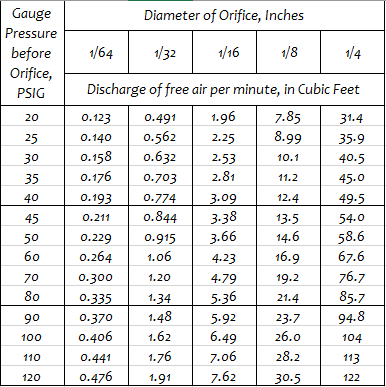## Discharge of Air Through an Orifice

My Application Engineer colleagues and I frequently use a handy table, called Discharge of Air Through an Orifice. It is a useful tool to estimate the air flow through an orifice, a leak in a compressed air system, or through a drilled pipe (a series of orifices.) Various tables and online calculators are available. As an engineer, I always want to know the ‘science’ behind such tables, so I can best utilize the data in the manner it was intended.The table is frequently found with values for pressures less than 20 PSI gauge pressure, and those values follow the standard adiabatic formula and will not be reviewed here.  The higher air pressures typically found in compressed air operations are of interest to us.

For air pressures above 15 PSI gauge the discharge is calculated using by the approximate formula as proposed by S.A. Moss. The earliest reference to the work of S.A. Moss goes back to a paper from 1906.  The equation for use in this table is-Where:For the numbers published in the table above, the values were set as follows-

C = 1.0,      p1 = gauge pressure + 14.7 lbs/sq. in,    and T1 = 530 °R (same as 70 °F)

The equation calculates the weight of air in lbs per second, and if we divide the result by 0.07494 lbs / cu ft (the density of dry air at 70°F and 14.7 lbs / sq. in. absolute atmospheric pressure) and then multiply by 60 seconds, we get the useful rate of Cubic Feet per Minute.

The table is based on 100% coefficient of flow (C = 1.0)  For well rounded orifices, the use of C = 0.97 is recommended, and for very sharp edges, a value of C = 0.61 can be used.

The table is a handy tool, and an example of how we use it would be to compare the compressed air consumption of a customer configured drilled pipe in comparison to that of the EXAIR Super Air Knife.  Please check out the blog written recently covering an example of this process.

If you would like to talk about the discharge of air through an orifice or any of the EXAIR Intelligent Compressed Air® Products, feel free to contact EXAIR and myself or one of our Application Engineers can help you determine the best solution.

Brian Bergmann
Application Engineer

Send me an email
Find us on the Web